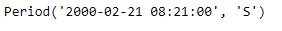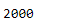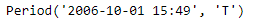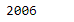# Python | Pandas Period.qyear

• Last Updated : 06 Jan, 2019

Python is a great language for doing data analysis, primarily because of the fantastic ecosystem of data-centric python packages. Pandas is one of those packages and makes importing and analyzing data much easier.

Pandas` Period.qyear` attribute return the fiscal year the Period lies in according to its starting-quarter. The year and the qyear of the period will be the same if the fiscal and calendar years are the same. When they are not, the fiscal year can be different from the calendar year of the period.

Attention geek! Strengthen your foundations with the Python Programming Foundation Course and learn the basics.

To begin with, your interview preparations Enhance your Data Structures concepts with the Python DS Course. And to begin with your Machine Learning Journey, join the Machine Learning - Basic Level Course

Syntax : Period.qyear

Parameters : None

Return : fiscal year

Example #1: Use `Period.qyear `attribute to find the fiscal year the period lies in for the given Period object.

 `# importing pandas as pd``import` `pandas as pd`` ` `# Create the Period object``prd ``=` `pd.Period(freq ``=``'S'``, year ``=` `2000``, month ``=` `2``,``                  ``day ``=` `21``, hour ``=` `8``, minute ``=` `21``)`` ` `# Print the Period object``print``(prd)`

Output :Now we will use the `Period.qyear` attribute to find the fiscal year

 `# return the fiscal year``prd.qyear`

Output :As we can see in the output, the `Period.qyear `attribute has returned 2000 indicating that the given period lies in the year of 2000.

Example #2: Use `Period.qyear` attribute to find the fiscal year the period lies in for the given Period object.

 `# importing pandas as pd``import` `pandas as pd`` ` `# Create the Period object``prd ``=` `pd.Period(freq ``=``'T'``, year ``=` `2006``, month ``=` `10``,``                            ``hour ``=` `15``, minute ``=` `49``)`` ` `# Print the Period object``print``(prd)`

Output :Now we will use the `Period.qyear` attribute to find the fiscal year

 `# return the fiscal year``prd.qyear`

Output :As we can see in the output, the `Period.qyear `attribute has returned 2006 indicating that the given period lies in the year of 2006.

My Personal Notes arrow_drop_up